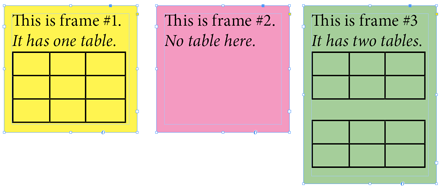Suppose your active document, doc, has three text frames. Then the `doc.textFrames` collection has exactly three elements and, of course,

`doc.textFrames.length == doc.textFrames.count() // == 3`

But it is misleading to put `length` and `count()` on an equal footing. In fact, these are not the same commands at all.

## Length

Let K denote any formal collection, that is, any DOM path that ends up in a collection property like `.stories`, `.words``.tables`, etc. For example, `K=doc.textFrames.everyItem().tables` is a formal collection which represents the tables of all text frames. The scripting subsystem treats K as a single `Tables` object and lets it enjoy all properties and methods of any collection. In particular, you can apply the `.everyItem()` operator to K and get a plural `Table` specifier, which lets you enter in sub-collections like `.cells`, `.pageItems`, and so on.

But here is the point: no matter how complex your formal collection is, `K.length` always returns a single integer, representing the overall number of elements available in that collection.Thus, if your document still has three frames which respectively contain one, zero, and two tables as illustrated above, then `K.length` (that is, `doc.textFrames.everyItem().tables.length`) will return the total number of available tables (3 = 1+0+2).

`K.length` is therefore a flat command that merges all individual results. It is strictly equivalent to a much longer, and much less efficient syntax: `K.everyItem().getElements().length` (!!)

## Count

Unlike `K.length`, `K.count()` considers separately the concrete collection(s) involved in K (in our example, `frame1.tables`, `frame2.tables`, `frame3.tables`). The command then gets the number of elements available in each collection and outputs an array of integers (instead of the overall sum), provided that several objects are visited:

```K = doc.textFrames.everyItem().tables;

// An array of three numbers is returned
// because there are three text frames.
// ---
alert( K.count() );  // => 1,0,2
```

Although poorly understood, the `count()` method is very powerful. In a way it allows a formal collection—which is not itself a plural specifier—to temporarily behave as a plural entity for counting purpose. Remember how the `doc.textFrames.everyItem().id` syntax works: it generates an array of IDs. But `doc.textFrames.everyItem().tables` is not an array of `Tables`, it's just a single, formal `Tables` collection. Thanks to `.count()`, we get access to the table count of each text frame upstream the `.tables` selector.

In other words,
`doc.textFrames.everyItem().tables.count()`
works as if the `TextFrame` object had a `tablesCount` property. It then returns:
`doc.textFrames.everyItem().tablesCount`
which is an array of `tablesCount` values.

Note. — If a single entity is selected upstream, then `K.count()` returns a single value and `K.length==K.count()`. A stable property is is that `K.length` represents the sum of the values returned in `K.count()`.

## ExerciseGiven a `Table` myTable formed of 6 cells, we notice that:

`myTable.cells.everyItem().texts.count()`
returns an array of six 1's: `[1,1,1,1,1,1]`

while

`myTable.cells.itemByRange(0,-1).texts.count()`
simply returns `6` (unique number.)

What does this tell us about `cells.itemByRange(0,-1)` vs. `cells.everyItem()`?